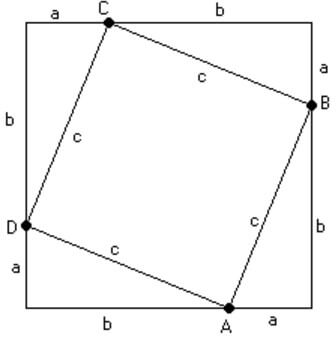# Proof of the Pythagorean Theorem

If we want to understand how the Pythagorean Theorem works, we have to understand certain mathematical concepts that are related to it and without which it would be complicated or even impossible to understand the proof of the Pythagorean Theorem.

Among the main points to keep in mind before understanding the proof of the Pythagorean Theorem are:

• A right triangle is that which has a right angle (90°) among its interior angles and the Pythagorean Theorem can only be applied to these geometric figures.
• Each side of a right triangle has a specific name; the longest side is given the name hypotenuse, while the remaining sides are called legs.

Based on these premises, we can quote the famous Pythagorean Theorem which states that “in any right triangle, the hypotenuse squared has the same value as the sum of the squares of both legs”.

hypotenuse2 = legA2 + legB2

To prove this premise, we’ll use a right triangle like that in the picture, where we will call each of the legs a and b and the hypotenuse c. From this triangle, we will make a square whose sides are the sum of both legs as shown below:Now, we see that the area of this square is equal to (a + b)2

We see that where the hypotenuses meet we have a new square with side c; in this way, the area of the larger square can be expressed as the sum of the area of square ABCD with the 4 right triangles that are shown in the figure.

Therefore, we have:

(a + b)2 = c2 + 2ab

To simplify this expression, we have to expand the binomial with a and b.

a2 + 2ab + b2 = c2 + 2ab

Now, we delete the term 2ab from both sides of the equation:

a2 + b2 = c2

Which proves the Pythagorean Theorem.